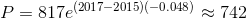# AP Calculus BC : Comparing Relative Magnitudes (exponential growth,logarithmic growth, polynomial growth)

## Example Questions

### Example Question #44 : How To Find Rate Of Change

A cylinder of height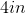and radius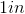is expanding. The radius increases at a rate of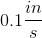and its height increases at a rate of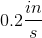. What is the rate of growth of its surface area?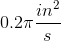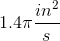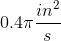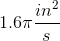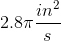Explanation:

The surface area of a cylinder is given by the formula: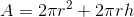To find the rate of growth over time, take the derivative of each side with respect to time: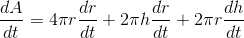Therefore, the rate of growth of surface area is: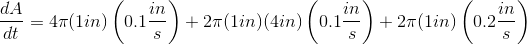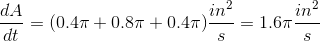### Example Question #1 : Comparing Relative Magnitudes (Exponential Growth,Logarithmic Growth, Polynomial Growth)

The rate of growth of the population of Reindeer in Norway is proportional to the population. The population increased from 9876 to 10381 between 2013 and 2015. What is the expected population in 2030?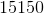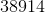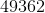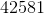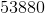Explanation:

We're told that the rate of growth of the population is proportional to the population itself, meaning that this problem deals with exponential growth/decay. The population can be modeled thusly: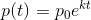Where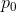is an initial population value, andis the constant of proportionality.

Since the population increased from 9876 to 10381 between 2013 and 2015, we can solve for this constant of proportionality: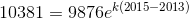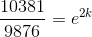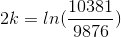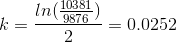Using this, we can calculate the expected value from 2015 to 2030: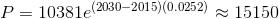### Example Question #2 : Comparing Relative Magnitudes (Exponential Growth,Logarithmic Growth, Polynomial Growth)

The rate of decrease due to poaching of the elephants in unprotected Sahara is proportional to the population. The population in one region decreased from 1038 to 817 between 2010 and 2015. What is the expected population in 2017?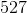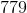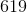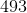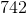Explanation:

We're told that the rate of growth of the population is proportional to the population itself, meaning that this problem deals with exponential growth/decay. The population can be modeled thusly:Whereis an initial population value, andis the constant of proportionality.

Since the population decreased from 1038 to 817 between 2010 and 2015, we can solve for this constant of proportionality: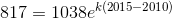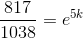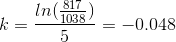Using this, we can calculate the expected value from 2015 to 2017: